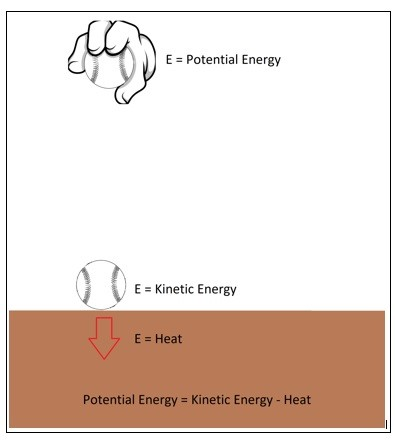# Intro to Thermodynamics

Thermodynamics is the study of energy. The name thermodynamics stems for the Greek words therme meaning heat and dynamics meaning power.  This represents the early efforts of converting heat into power.  Today thermodynamics is the study of all aspects of energy transformation.  This includes power generation, refrigeration, as well as relationships between the property of matter.

### Conservation of Energy

One of the fundamental laws of nature is the conservation of energy principle.  What the conservation of energy states is that during an interaction, energy can change from one form to another, but the total amount of energy must remain the same.  What this means is energy cannot be created or destroyed.  Energy however can change forms.

##### Example

Let’s say you are holding a ball a certain distance $h$ from the ground.  At this point the ball will have potential energy of,

$E = mgh$

$m$ = mass

$g$ =  gravitational constant

However, once you release that ball its potential energy will convert to kinetic energy.

$E=\frac{1}{2}mv^2$

$v$ = velocity

Once the ball hits the ground all of its stored potential energy would have become kinetic energy.  However, the ball will bounce causing the kinetic energy to become potential energy again.  Since energy cannot be created or destroyed this process should continue indefinitely.  In reality the ball will stop bouncing.  This is due to energy being lost to an unusable form of energy either through heat due to friction, air drag, or from the actual impact of the ball when it hits the floor.  However, it is important to realize that this lost energy is not energy that was destroyed it was just energy that was transformed into an unusable form of energy.### Overview of the Laws of Thermodynamics

The first law of thermodynamics is an expression of the conservation of energy principle where it states that energy is a thermodynamics property.

The second law of thermodynamics states that energy has quality as well as quantity, where the process occurs in a direction of decreasing quality of energy.  An example of this would be if you had left a hot plate of food on the table.  That plate of food will eventually cool to room temperature.  It will not, however, return to its original temperature by itself.  Instead to return the plate of food to its original temperature you will need add energy to it that wasn’t originally there.

### Application of Thermodynamics

In nature all activities where there is an interaction between matter energy will have a relation to thermodynamics.  You’re heart pumping the blood inside your body and the energy conservation trillion of cells in your body can all be described by thermodynamics.  As a matter of fact the formation of the universe can be described by thermodynamics.  In fact if the laws of thermodynamics were to stop working, the whole universe would essentially die.

As an engineer, we seek to find a way to use the laws of thermodynamics to transform energy into some form of work. This is done inside power plants to create the electricity that powers your house.  Thermodynamics is also used to design your car’s engine.  It is also used to cool your food in your refrigerator, or to cool or heat your house with a heat pump.  These are just a few examples of applications that use thermodynamics within their design, there are in fact many more, which will be discussed on other pages within this website.

Next